# Problem: A block lies on a plane raised an angle  from the horizontal. Three forces act upon the block:, the force of gravity; , the normal force; and , the force of friction. The coefficient of friction is large enough to prevent the block from sliding (Intro 1 figure).Consider coordinate system a, with the x axis along the plane. Which forces lie along the axes?

###### FREE Expert Solution

The normal force, Fn, points in the positive y-axis.

91% (60 ratings)###### Problem Details

A block lies on a plane raised an angle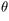from the horizontal. Three forces act upon the block: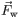, the force of gravity;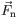, the normal force; and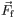, the force of friction. The coefficient of friction is large enough to prevent the block from sliding (Intro 1 figure).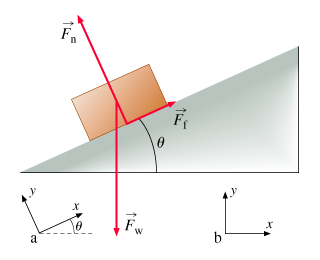Consider coordinate system a, with the x axis along the plane. Which forces lie along the axes?